# Ohm's Law

## SPECIFIC OBJECTIVES

• To test Ohm's Law (V = Ri) by plotting V vs. i for a wire and to determine the resistance (R) of the wire .
• To test the resistivity law (R =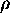L / A) by plotting R vs. L for a wire and to determine the resistivity () for the material of which the wire is made.
• To become familiar with the voltmeter-ammeter method for measuring resistance.

## EQUIPMENT

Board with ten 1-meter lengths of a wire mounted between binding posts, power supply, analog voltmeter, multimeter used as ammeter and later as an ohmmeter, hook-up wire, micrometer.

## GLOSSARY

• The ELECTRICAL RESISTANCE (R) of a device is defined to be the ratio of the voltage (V) across the device to the current (i) through the device R = V / i. The unit of resistance, ohm (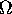, the Greek letter capital omega), is then defined to be the resistance when one volt exists across and one amp flows through the device,= V/A.
• OHM'S LAW a condition of some materials where the resistance is constant regardless of the voltage applied across the device. For materials that obey Ohm's Law (some do not), a plot of voltage vs. current yields a straight line whose slope is the resistance of the material.

## BACKGROUND

For some materials, the resistance is constant no matter how much voltage is applied across it. These materials are said to obey Ohm's Law. Since the resistance (R) is constant, a plot of voltage (V) vs. current (i) yields a straight line for these materials. Notice that resistance is always the ratio of voltage across a device to the current through the device. But the resistance is constant only for those materials that obey Ohm's Law. For this experiment, we will be studying a material known to obey Ohm's Law.

Ohm's Law suggests a method for measurement of resistance. If a voltmeter is used to measure the voltage (V) across an unknown resistance (R), and an ammeter is used to measure the current (i) through the same unknown resistance, then R would be given by R = V/i. The two measures of V and i should, of course, be made simultaneously. Some further consideration of the voltmeter-ammeter method (V-A method) reveals that there is inherent error involved. Consider the two circuits (cct.) shown below.In cct. 1, the ammeter (A) reads the true current (i) through the unknown resistance (R) but, the voltmeter (V) reads the voltage across both A and R. Thus, the V-reading is larger than that which is required so the calculated R would be in error i.e., too large:
Rcalc = (VR + VA) / i
Ammeters are generally designed to have a small internal resistance (20 ohms) so, if R is large, then the error (Rcalc - R) would be small i.e., practically all of the V-measure is across R, and VA can be neglected compared to VR.

In cct. 2, the voltmeter reads the true voltage across R but now the ammeter reads the current (i) through both the voltmeter and R. Thus, the measured current is larger than that which is required so the calculated R would be in error, i.e., too small:

Rcalc = V / (iR + iV)
Voltmeters are generally designed to have a large internal resistance (megaohms) so, for practical purposes, nearly all of the i-measure flows through R, very little current flows through the high-resistance voltmeter, and iV can be neglected compared to iR. Considering the role of internal meter resistance, cct. 1 is better (smaller error) if R is large whereas, cct. 2 is preferable if R is small.

The Ohmmeter and Wheatstone Bridge provide two other methods for the determination of resistance. The ohmmeter is not generally a precision device but is adequate in many electronic applications. The Wheatstone Bridge can be refined to provide precision resistance measures as might be required in the use of such as a resistance thermometer.

A property of electrical resistance which may need to be considered in some cases is that it depends on temperature to some extent. The resistance (R) is given by: R = Ro(1 +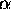T), where T is the temperature, Ro is the resistance at 0oC andis the TEMPERATURE COEFFICIENT OF RESISTANCE. Fortunately,is small for some metals such as copper and aluminum but can be relatively large for others. The latter are useful as resistance thermometers. Recalling that the time rate of heat production (Power = P) in a resistance (R), carrying a current (i) is given by

P = R i2.
the temperature dependency of resistance may or may not be especially relevant depending on whether i is large or small.

Ohmic materials or devices are those which obey Ohm's Law but there are non-ohmic things to which the law cannot be properly applied. While Ohm's Law does have wide applicability, it does also have limitations.

The RESISTIVITY LAW is that R =L / A where R is the resistance of a wire of length L, cross-sectional area A and made of a material whose RESISTIVITY is. Logically, R is proportional to L, and R is also proportional to l/A sois a proportionality constant which depends only on the material of which the wire is made and not on the wire's length, cross-sectional area, or shape.

One test of the resistivity law could be to measure the resistance (R) of various lengths (L) of a certain gauge (diameter or cross-sectional area) and kind (material) of wire. A linear plot of R vs. L should reveal that R is prportional to L and, according to the law, the slope of this plot should be/ A. If the diameter of the wire is measured, from which the area (A) of the wire may be calculated, then from the slope of R vs. L,could be found.

## PROCEDURE

1. Read the precautions for using a multimeter as a current meter. Bear in mind that the colors of the lead wires in this experiment will differ from the colors of the probes in the precautions.
2. Adjust the power supply to zero volts and connect the circuit as shown below leaving the voltmeter probe (P) disconnected at this time.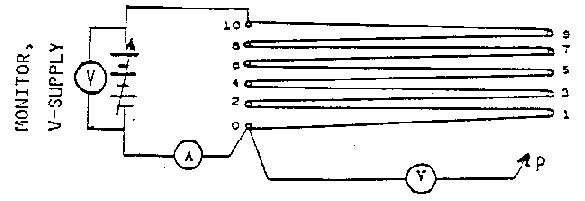### Ohm's Law

3. Connect the voltmeter probe (P) to terminal 10 and check the wiring to see that the voltmeter (V) will read the voltage across the entire 10-meter length of wire while the ammeter (A) reads the current through the wire. Set the ammeter on DCA function and on the 10 A range. Set the voltmeter on DCV function and use the 15V range. Turn the power supply on and slowly increase the voltage until A and V begin to show readings. It is now a matter of reading A and V simultaneously to provide data for the V vs. i plot. The range for i should be from about 0.2 A to about 0.8 A and i should not exceed 1.0 A. Give some thought to the criteria involved and choose the current increments to provide an appropriate number and distribution of points. Record this V vs. i data. In attempting to read voltage and current simultaneously, perhaps two partners could each read a meter on some signal.

### Resistivity Law

4. Adjust the power supply to zero volts and remove the probe from the terminals. Increase the power supply voltage until the current through the wire is about 0.75 A. After a few minutes, when thermal equilibrium is reached, the current should stabilize. Touch the probe to the lm terminal and read and record voltage and current simultaneously. Continue this procedure until the 2m, 3m...10m. terminals are used recording L, V, and i at each step.
5. Use a Vernier micrometer to measure the diameter of the wire in several places. Do not stretch the wire in measuring the diameter. Two measurements on each side-wire should be adequate. Also, close the micrometer, read it, and make a zero correction on subsequent readings. A reliable average diameter is needed to ensure that the calculated area is representative of the wire.

### Ohmmeter Method

6. Read the precautions for using a multimeter as an ohmmeter. Bear in mind that the colors of the lead wires in this experiment will differ from the colors of the probes in the precautions.
7. Use the ohmmeter function of a multimeter to measure the resistance of the 10-m length of wire. To avoid possible damage to the meter, the wire must not be connected to the power supply when the ohmmeter is used.
8. Short out (touch together) the ohmmeter lead wires to read and record the lead-wire resistance.

## ANALYSIS

### Ohm's Law

1. Plot V vs. i, read R from the slope, and calculate### Resistivity Law

2. Calculate the propagated error on R from the reading errors on V and i for each line in your data table.
3. Plot R vs. L, read(not equal to the slope, but related simply to it), and calculate R.

### Ohmmeter Method

4. How does the ohmmeter value of R compare to the resistance of 10 m of the wire as found from your plot of V vs. i? Also, compare to that found from R vs. L. (Show how you found these latter R's). Note that this comparison involves the ohmmeter method vs. the V-A method. Does it seem that the previous ohmmeter reading should or should not be corrected for the lead-wire resistance? Explain. In other words, the ohmmeter method gives the resistance of both the board AND the lead wires; do the other methods measure the resistance of just the board, or do they also measure the lead wire resistance. If all three methods measure the same thing, no correction is nesscessary.
5. There are now available three different measures (two plots and ohmmeter) of the resistance of 10 m of the wire. List these three R-values and calculate the mean and standard deviation on the mean (SDOM). Now what do you believe the resistance of your 10-m length to be, in the form of R ±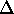R? (The point is, that after all this measuring, R10 is still not exactly known! So - it must be acknowledged that some measurement error exists in all experimental work.)
6. Identify at least two sources of random (statistical) error.
7. Identify at least two sources of systematic error.
8. Bonus: Look up the resistivity of the wire in the CRC Handbook of Chemistry and Physics and identify the material.

## CONCLUSION

Summarize what you learned today (not what you did).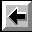Back to the Electricity and Magnetism Manual